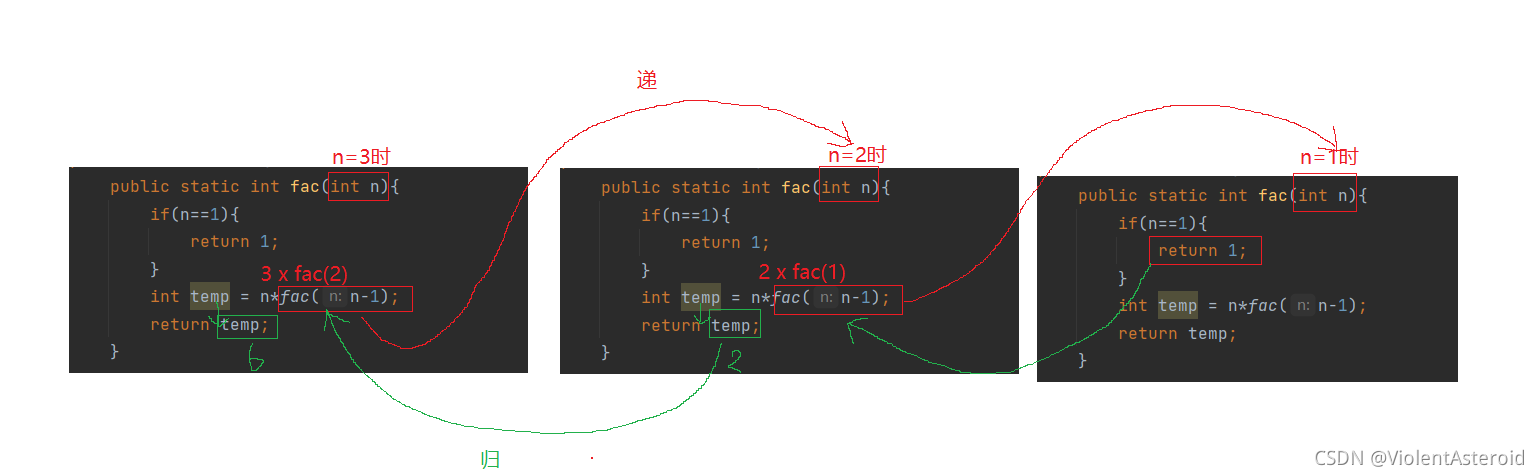﻿ Java中方法的使用、重载与递归的详细介绍_java_脚本之家
java# Java中方法的使用、重载与递归的详细介绍

## 一.方法的基本使用

### 2.方法基本语法

``` // 方法定义
public static 方法返回值 方法名称（参数类型 形参){
方法体代码;
return 返回值;
}

public static void main(String[] args) {
// 方法调用
返回值变量 = 方法名称 (实参);
}```

```public class TestDemo {
// 方法定义
public static int Add(int x,int y){
int sum = x+ y;
return sum;
}

public static void main(String[] args) {
//方法的调用
}
}```

1.方法定义时, 参数可以没有。每个参数要指定类型

2.方法定义时, 返回值也可以没有, 如果没有返回值, 则返回值类型应写成 vo

3.方法定义时的参数称为形式参数（形参），方法调用时的参数称为实际参数（实参）

4.方法的定义必须在类之中, 代码书写在调用位置的上方或者下方都可以

5.所有程序的入口：main函数

```public class Test1 {
public static void swap(int a,int b){
int temp = a;
a = b;
b = temp;
}

public static void main(String[] args) {
int a = 10;
int b = 20;
System.out.println("交换实参前："+a+" "+b);
swap(a,b);
System.out.println("交换实参后："+a+" "+b);
}
}```## 二.方法的重载

### 1.重载的作用

```public class Test2 {
public static int addInt(int a, int b){
int sum = a+b;
return sum;
}

public static double addDouble(double a,double b){
double sum = a+b;
return sum;
}

public static void main(String[] args) {
double a = 10.5;
double b = 20.5;
}
}``````public class Test2 {
public static int add(int a, int b){
int sum = a+b;
return sum;
}

public static double add(double a,double b){
double sum = a+b;
return sum;
}

public static void main(String[] args) {
double a = 10.5;
double b = 20.5;
}
}```### 2.重载的使用规则

1.方法名相同

2.方法的参数不同(参数个数或者参数类型)

3.方法的返回值类型不影响重载

## 三.方法的递归

### 2.递归执行过程分析

```public class Test3 {
public static int fac(int n){
if(n==1){
return 1;
}
int temp = n*fac(n-1);
return temp;
}

public static void main(String[] args) {
System.out.println(fac(3));
}
}```### 3.递归练习

#### 1.按顺序打印一个数字的每一位

```public class Demo1 {
public static void print(int n){
if(n>9){
print(n/10);
}
System.out.print(n%10+" ");;
}

public static void main(String[] args) {
int n = 1234;
print(n);
}
}```#### 2.递归求 1 + 2 + 3 + ... + 10

```public class Demo2 {
public static int func(int n){
if(n!=0){
n += func(n-1);
}
return n;
}
public static void main(String[] args) {
System.out.println(func(10));
}
}```#### 3.返回组成它的数字之和

```public class Demo3 {
public static int func(int n){
if(n<10){
return n;
}
return n%10+func(n/10);
}

public static void main(String[] args) {
System.out.println(func(1792));
}
}```#### 4.求斐波那契数列的第 N 项```public class Demo4 {
public static int fib(int n){
if(n==1 || n == 2){
return 1;
}else{
return fib(n-1)+fib(n-2);
}
}

public static void main(String[] args) {
System.out.println(fib(1));
System.out.println(fib(2));
System.out.println(fib(3));
System.out.println(fib(4));
System.out.println(fib(123456));
}
}``````public class Demo4 {

public static int fib2(int n){
if(n==1 || n==2){
return 1;
}
int f1 = 1;
int f2 = 1;
int f3 = 0;
for (int i = 3; i <= n; i++) {
f3 = f1 + f2;
f1 = f2;
f2 = f3;
}
return f3;
}

public static void main(String[] args) {
System.out.println(fib2(1));
System.out.println(fib2(2));
System.out.println(fib2(3));
System.out.println(fib2(4));
System.out.println(fib2(40));
}
}```